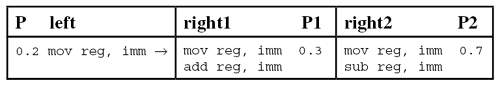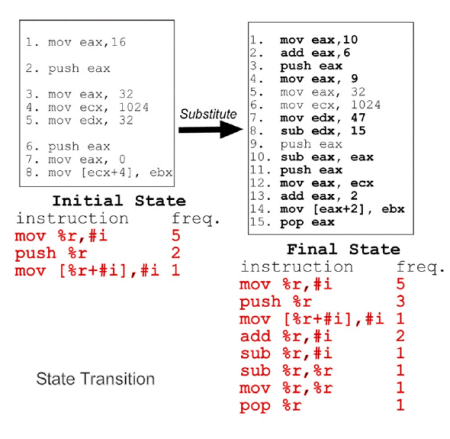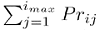# Metamorphic authorship recognition using Markov models

2008-05-01

Editor:

Abstract

Automated code morphing techniques can make malware recognition difficult. In this article researchers at the University of Lafayette propose a method that can be used to decide whether a binary is a variant of a known item of metamorphic malware by treating the morphing engine as an author.

Automated code morphing techniques can make malware recognition difficult . Morphed malware can be detected by recognizing invariant runtime behaviours, or by first normalizing the programs to remove the variations introduced by the morphing engine . While effective, these methods are computationally expensive to apply to every object scanned.

We propose a fast method that can be used to decide whether a binary might be a variant of a known item of metamorphic malware. The key idea is to treat the morphing engine as an author, and then use its morphing characteristics to decide whether a suspect program is a variant of the original, or ‘Eve’ version.

## Introduction

Since the time of the Morris worm it has been suggested that it may be possible to trace a program to its authors by noting features of the program that are likely to fit the output profile of what those authors may generate . But morphed programs are the output of a certain kind of ‘author’ – the morphing engine. This observation leads us to ask: is it possible to recognize morphed malware by virtue of recognizing its author in the form of the morphing engine? We propose a method for recognizing metamorphic malware – which may generate successive transformations of itself – using mechanisms similar to those used in determining the authorship of ordinary text.

Our approach starts with a model of the metamorphic engine as a probabilistic generator of text. We employ a simple model for closed-world probabilistic instruction-substituting, metamorphic malware.

With the model in hand, some method of selecting authorship features is needed, and a method is required for matching the expected features to those found in a program whose authorship is in question. We propose to use instruction frequency vectors, or IFVs, as the program abstractions to compare. An a priori computable transition matrix is used to construct predicted IFVs for different generations of the metamorphic malware. This transition matrix is computed using a model of the metamorphic engine. The predicted IFVs can then be compared to the IFV of a suspect program to determine whether it is a descendant of the Eve. Markov theory  is used to formalize the frequency vector comparison approach.

## Probabilistic author model

We are interested in recognizing variants of closed-world probabilistic instruction-substituting metamorphic malware. This class of malware carries a metamorphic engine that uses a fixed, finite set of transformation rules, each of which maps an instruction (the left-hand side) to a set of possibly larger code segments (the right-hand sides).

An example of this type of rule set appears in Figure 1. In the example there are two rules, which for comparison purposes in the analysis below have identical left-hand sides. When the left-hand sides are found, the rule is fired with the probability of 0.2. There are two right-hand sides which are selected at probabilities 0.3 and 0.7, respectively.Figure 1. Probabilistic instruction substitution.

These rules are typically carried as data in the malware code. They are used by the engine, perhaps along with other transformations, to substitute occurrences of the left-hand sides of the rules probabilistically with one of their corresponding right-hand sides. The probabilities are assumed to be fixed and exactly learnable from the description of the engine. While this is a simple model of metamorphic malware, it captures the essential elements of probabilistic substitution.

Metamorphic malware gives rise to variations through the generation of descendants. Let M denote some metamorphic engine and (M, x) a malicious program using M to transform its own code. The set of all programs (M, y) into which (M, x) can possibly be transformed at the end of a run of M are called the ‘first-generation descendants of (M, x)’. More generally, for positive integer n, an (n + 1)-generation descendant of (M, x) is a first-generation descendant of an nth-generation descendant of (M, x). The descendants of a given (M, x) are often called variants of each other.

## Predicting feature frequency vectors

A set of features of a given program must be used to determine whether it has been authored by a given morphing engine. Ideally, the set of features selected is such that the idiosyncrasies of the authoring program – its specific generation characteristics – can be detected. In this paper we use instruction forms, which are assembly-level instructions in an abstract form. Depending upon the implementation chosen, these could use merely the operations themselves (i.e. without parameters or prefixes), or employ instruction ‘templates’ that keep parameter counts and indexing modes but ignore all other details. As will be shown, matching involves comparing the frequencies of such features against the frequencies we can expect from the metamorphic malware.

Let P denote a program and n the number of distinct instructions occurring in P. The instruction frequency vector of P, denoted IFV (P), is the n-tuple of pairs, each of which consists of an assembly operation and the frequency (or count) of that operation in P. For example, consider a code segment s whose operations (ignoring all parameters and prefixes in this case) follow the sequence: mov, add, push, mov, mov, add, pop. Then:

IFV (s) = [(mov, 3), (add, 2), (push, 1), (pop, 1)]

For any sequence of generations of malware created by a probabilistic morpher, the IFVs will evolve in a predictable way. Our model of probabilistic morphing does not consider any previous transformations when selecting a transformation rule to apply (i.e. it has no ‘memory’). It can therefore be treated as a first-order Markov process, and the sequence of descent is a Markov chain. Each application of a transformation rule by the engine serves to transform the IFV for the program. Figure 2 illustrates an example of such a transformation using instruction ‘templates’ as the instruction forms being used. A new generation is created when the metamorphic malware applies one or more such rules probabilistically on one of its variants. Producing such a new generation is, in our Markov model, taken to be a state transition. The IFV of the original variant (our ‘Eve’) is the initial state.Figure 2. IFV transition induced by metamorphic transformation.

Utilizing Markov theory has several advantages. It provides clear formalization of the computations needed to predict the evolution of IFVs as the metamorphic engine produces new generations. Moreover, Markov theory has identified certain interesting classes of chains and ways of using a chain’s transition matrix to infer useful information about the process it represents. Two of these results suggest clearly how and when the IFV transition matrix (whose computation is discussed further below) can be used to assist in the detection of descendants of a given malware variant.

### Distribution prediction using the successive powers of the transition matrix

Typically, Markov chains are started in a state determined by a probability distribution on the set of states, called a probability vector. Let u denote a probability vector which holds the initial probabilities of a malware’s IFV. Then Tn is the nth power of T, and Tij(n) is the i, j-th entry of Tn. The powers of T are known to give interesting information about the evolution of these IFVs from one malware generation to the next: for any positive integer n, Tij(n) gives the probability that the chain, starting in state si, will be in state sj after n steps. More generally, if we let u(n) = uTn, then the probability that an nth-generation malware descendant has IFV

### Convergence towards a stationary state distribution

For every transition matrix T of a Markov chain with a finite space, there exists at least one stationary distribution, i.e. a row vector s satisfying s = sT. Furthermore, if T is irreducible and aperiodic, then it has a unique, a priori computable stationary distribution given by lim Tn = 1s, where 1 is a column vector all of whose entries equal 1.

Hence, for a piece of malware whose starting probability distribution on the set of IFVs happens to be a stationary distribution for its engine’s IFV transition matrix, the corresponding states of the elements of every generation of descendants will be distributed as indicated by s.

## Computing the IFV transition matrix

Let I = {I1, I2, ..., IM} denote the set of valid instruction forms for the considered computing platform. Let T denote a finite set of k productions of the form:

li → {(Prij , rij) : 1 ≤ jimax)}

where liI, rijI+, I+ is the set of all non-empty strings of elements of I, imax is the number of right-hand sides indexed by i, and Prij is the probability of use of the sequence of instructions rij to substitute an occurrence of li in the program being transformed. In order to allow the engine to choose whether or not to transform an occurrence of li, we require that exactly one of the rij be identical to li.

We also require that the identityholds for each production and that two different productions do not have identical left-hand sides.

Using the expression of the engine’s productions, we can compute:

1. The probability that an arbitrary instruction i will be transformed by the engine into an arbitrary number xj of some instruction j.

2. The probability that xi instances of an arbitrary instruction i will be transformed by the engine into an arbitrary number xj of some instruction j.

3. The probability that a code segment with an IFV v will be transformed by the engine into an arbitrary number xj of some instruction j.

4. The probability that a code segment with an IFV v will be transformed by the engine into a code segment with an IFV v’.

The computation of the probabilities of step 2 requires individual substitutions performed by an engine on a variant to be mutually independent. Computation of each of these probabilities is hence NP-complete in general, since they each require a solution for an instance of the SUBSETSUM problem.

SUBSETSUM takes as input a set of integers and asks whether there is a subset of those whose elements sum to a target number. An approximate solution to SUBSETSUM may hence be needed here to compute these probabilities approximately.

Successful completion of step 4 yields the IFV transition matrix of the malware using the probabilistic instruction-substituting engine represented by the matrix. Each element of the matrix represents a state transition from one IFV to another.

## Decision procedure

A malware detector can use the IFV transition matrix to implement a fast decision procedure for determining whether a given program is a descendant of the Eve. Let D0 denote the initial distribution vector of IFVs for the malware. Hence, if we wish to recognize the descendants of a malware variant Eve, then we set all of the components of D0 to 0 except for that representing the IFV of the Eve. For any positive integer n, compute the vector Dn = D0T, where T is the engine’s induced IFV transition matrix. Deciding membership in nth-generation descendants of the Eve would consist of the following steps:

1. Disassemble the suspect program

2. Abstract the resulting assembly program into the sequence s of instruction forms

3. Extract the IFV of s

4. If ( Dn[IFV (s)] ≠ 0 )

then s is an nth generation descendant of Eve

else s is not an nth generation descendant of Eve

The expression Dn[IFV (s)] represents the component of Dn that corresponds to IFV (s). Since our goal is to decide membership approximately in the various generations of descendants of a given Eve, we only need to compute and use T to determine the IFV distribution vectors Dn, up to a chosen constant value n.

## Optimizations and approximations

It is possible for malware to have no upper bound on growth, and so in theory there may be no fixed upper bound for storing counts within the IFV. Hence, it is expected to be necessary to impose an upper bound on the size of this set while limiting, as much as possible, the deterioration of the predictive and classification power of the transition matrix. Moreover, without due care in modelling the features, the transition matrix may become too costly to construct or store. Thus, in practice, some optimizations and approximations will frequently be desirable so that the size is reduced.

Possible optimizations and approximations include, but are not limited to: (1) reducing the size of the instruction set by abstracting an assembly language instruction to its opcode mnemonic or by ignoring register names and variable values; (2) imposing an upper bound on the possible frequency of each individual instruction; (3) imposing an upper bound on the value held in any of an IFV’s components; (4) abstracting the range of possible frequencies of each instruction to an imprecise scale (e.g. ‘low’, ‘medium’, and ‘high’); and (5) removing from the instruction set those instructions which are as likely to appear in a malicious program as they are to appear in a benign one.

One optimization that may be used in practice to reduce the size of an engine’s induced transition matrix is first to pick a threshold t on individual opcode frequencies. If the frequency of an opcode in a code segment P is below the threshold, then the component of the IFV (P) that corresponds to that opcode is set to 0, else it is set to 1. Then, only a feasible count of instruction forms are considered relevant. If, say, only 10 are selected, then the IFV could be encoded using a vector of 10 binary numbers.

## Conclusion and further work

This paper brings to bear results in authorship determination of (human) documents on the problem of determining variants of a piece of metamorphic malware. After all, a metamorphic variant is a machine-authored (more accurately, machine-transformed) program.

The proposed approach suffers several limitations. The basic IFV transition matrix may grow too large for us to use in practice, and abstracting the matrix to optimize its size may cause a loss in the predictive power of the matrix. Furthermore, the probabilities of use may not be available. This may be remedied by estimating them from a corpus of descendants of a given malware Eve. Unfortunately, estimated probabilities may not be precise, resulting in a bias in the predictive power of the transition matrix.

We are currently investigating the possibility of extending the approach to general probabilistic metamorphism where arbitrary probabilistic transformations are employed by a metamorphic engine. It may be possible to capture the evolution of the instruction distribution of this type of malware as it transforms its own code.

### Bibliography

 Ször, P. The Art of Computer Virus Research and Defense. Symantec Press, Addison Wesley Professional, 1st ed., 2005.

 Walenstein, A.; Mathur, R.; Chouchane, M.R.; Lakhotia, A. Constructing malware normalizers using term rewriting. Journal in Computer Virology. 2008.

 Spafford, E.H.; Weeber, S.A. Software forensics: Tracking code to its authors. Computers & Security, vol. 12, pp. 585–595, December 1993.

 Meyn, S.P.; Tweedie, R.L. Markov Chains and Stochastic Stability. London: Springer-Verlag, 1993.

## Latest articles:

### Nexus Android banking botnet – compromising C&C panels and dissecting mobile AppInjects

Aditya Sood & Rohit Bansal provide details of a security vulnerability in the Nexus Android botnet C&C panel that was exploited to compromise the C&C panel in order to gather threat intelligence, and present a model of mobile AppInjects.

### Cryptojacking on the fly: TeamTNT using NVIDIA drivers to mine cryptocurrency

TeamTNT is known for attacking insecure and vulnerable Kubernetes deployments in order to infiltrate organizations’ dedicated environments and transform them into attack launchpads. In this article Aditya Sood presents a new module introduced by…

### Collector-stealer: a Russian origin credential and information extractor

Collector-stealer, a piece of malware of Russian origin, is heavily used on the Internet to exfiltrate sensitive data from end-user systems and store it in its C&C panels. In this article, researchers Aditya K Sood and Rohit Chaturvedi present a 360…

### Fighting Fire with Fire

In 1989, Joe Wells encountered his first virus: Jerusalem. He disassembled the virus, and from that moment onward, was intrigued by the properties of these small pieces of self-replicating code. Joe Wells was an expert on computer viruses, was partly…

### Run your malicious VBA macros anywhere!

Kurt Natvig wanted to understand whether it’s possible to recompile VBA macros to another language, which could then easily be ‘run’ on any gateway, thus revealing a sample’s true nature in a safe manner. In this article he explains how he recompiled…

We have placed cookies on your device in order to improve the functionality of this site, as outlined in our cookies policy. However, you may delete and block all cookies from this site and your use of the site will be unaffected. By continuing to browse this site, you are agreeing to Virus Bulletin's use of data as outlined in our privacy policy.